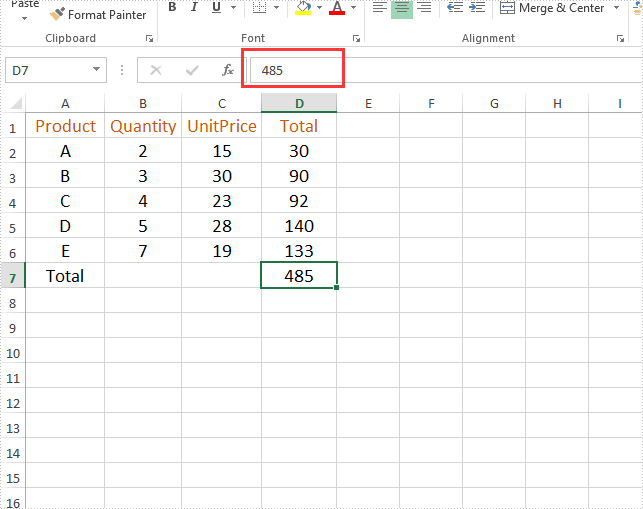# Java remove the formulas but keep the values on Excel worksheet

This article will demonstrate how to use Spire.XLS for Java to remove the formulas but keep the values on the Excel worksheet.

Firstly, view the original Excel:```import com.spire.xls.*;

public class Test {
public static void main(String[] args) throws Exception {

String inputFile = "Sample.xlsx";
String outputFile="output/removeFormulasButKeepValues_result.xlsx";

//Create a workbook.
Workbook workbook = new Workbook();
//Loop through worksheets.
for (Worksheet sheet : (Iterable) workbook.getWorksheets())
{
//Loop through cells.
for (CellRange cell : (Iterable) sheet.getRange())
{
//If the cell contains formula, get the formula value, clear cell content, and then fill the formula value into the cell.
if (cell.hasFormula())
{
Object value = cell.getFormulaValue();
cell.clear(ExcelClearOptions.ClearContent);
cell.setValue(value.toString());
}
}
}
//Save to file
workbook.saveToFile(outputFile, ExcelVersion.Version2013);
}
}
```

Output: